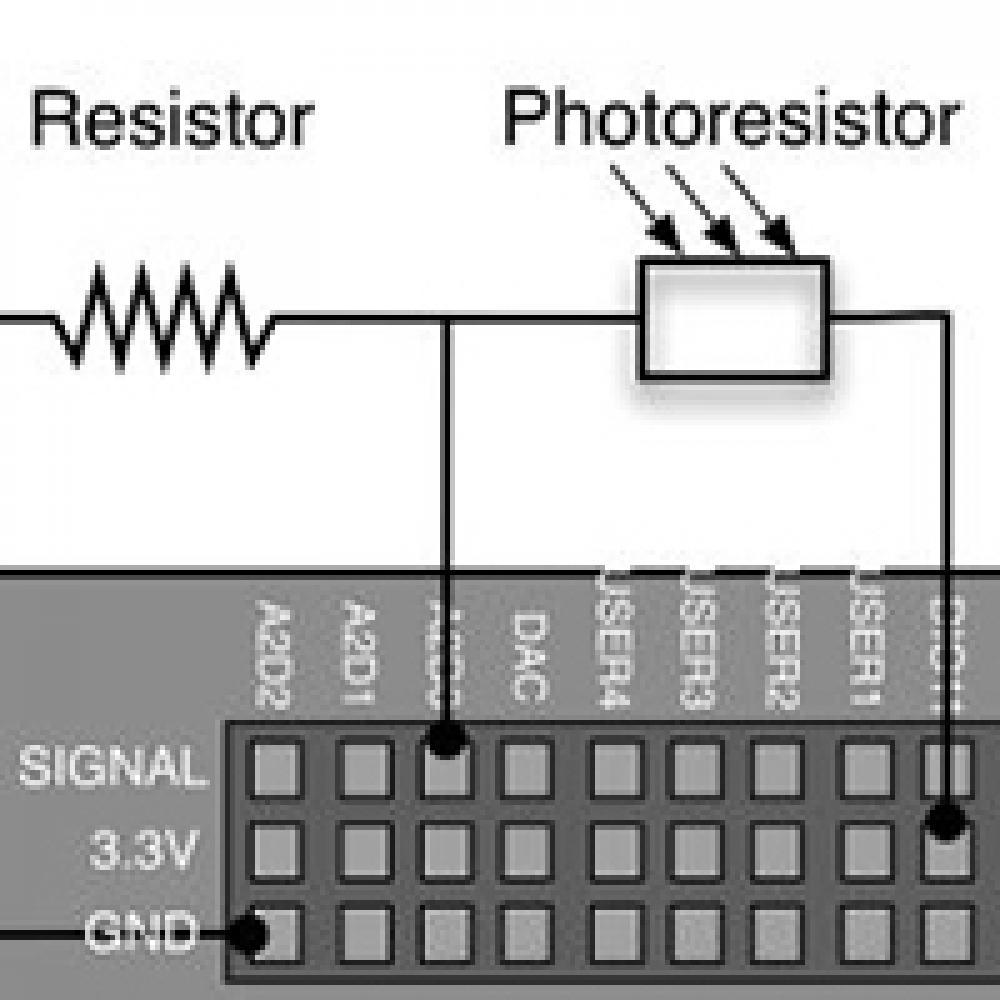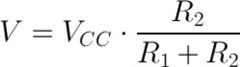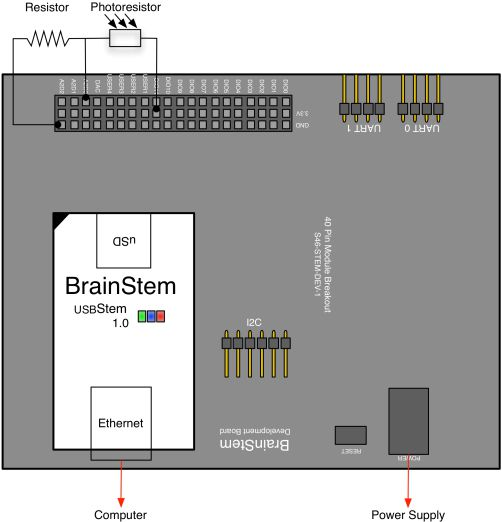# Reading a Photoresistor Asynchronously blog post

2009 December 25## OVERVIEW

Photoresistors are used in many applications because they either increase or decrease in resistance depending on the amount of light.  A common application for photoresistors is the triggering of an event when the light passes a certain point.  This example demonstrates the use of a Photoresistor to toggle the User LED.

BrainStem application examples require the BrainStem Support package.

## THE SETUP

There are several hardware components needed for this example:

• 40-Pin EtherStem 1.0 Module
• 40-Pin Development Board
• Ethernet Cable
• Power Supply
• Photoresistor
• Resistor

## PHOTORESISTOR

A photoresistor is a sensor whose resistance varies with light intensity.  Most photoresistors decrease in resistance as the light intensity increases.  Typically, the resistance must be converted to a voltage so that an A2D converter can measure it.  A voltage divider circuit is the easiest way to convert a resistance to a voltage. A voltage divider is just two resistors in series connected between a voltage supply and ground.  If R1 is connected to the voltage supply and R2 is connected to ground then the voltage at the junction between the two resistors is:If R1 is the photoresistor, the voltage will increase with increasing light intensity.  If R2 is the photoresistor, the voltage will decrease with increasing light intensity.

## CONFIGURATION

Connect the BrainStem to the development board with a power supply connected and the Ethernet cable connecting the Stem to the host computer.  Connect the Photoresistor to the 3.3V output and to the A2D pin [A2D0 for this example].  Connect a resistor in series between the Photoresistor and ground.Detailed ViewWiring Diagram

## READING ANALOG VOLTAGE ASYNCHRONOUSLY WITH REFLEX

To achieve an asynchronous state a Timer class is used.   The Expiration entity of the Timer class sets the length of time the Timer waits before executing.  When the Timer executes, the voltage of the analog pin is read using the Voltage entity of the Analog class.  With the Voltage entity, the voltage of the analog pin can be stored in a variable that can then be compared to a threshold.  The System class is used to change the state of the User LED, with the LED entity, depending on the comparison between the value of the analog pin and the threshold.  The Timer class is then used to initialize the Timer again, creating an asynchronous loop.

 1 2 3 4 5 6 7 8 9 10 11 12 13 14 15 16 17 18 19 20 21 22 23 24 25 26 `#include `   `#define DELAY 100000` `#define THRESHOLD 15000`   `a40PinModule stem;`   `reflex mapEnable()` `{` `  ``stem.timer.setExpiration(DELAY);` `} ``// end of mapEnable`   `reflex timer.expiration()` `{` `  ``unsigned ``short` `bPhotoState;`   `  ``stem.analog.getVoltage(bPhotoState);`   `  ``if` `(bPhotoState <= THRESHOLD) {` `    ``stem.``system``.setLED(``true``);` `  ``} ``else` `{` `    ``stem.``system``.setLED(``false``);` `  ``}`   `  ``stem.timer.setExpiration(DELAY);` `} ``//end of timer`